# Classroom Case Studies, Grades 6-8 Part D: More Problems That Illustrate Algebraic Thinking (25 minutes)

## Session 10: 6-8, Part D

At the 6-8 grade level, there are many problems that prepare students for their later work in algebra. There is some evidence that more students are, in fact, taking a formal course in algebra in the eighth grade. The problems included in this session, however, may be used in courses both before and during students’ first formal course in algebra. Note 7

For each of the problems below, answer the following questions:

 a. What algebraic content is in the problems? b. What content does it prepare students for later? c. How does this content relate to the mathematical ideas in this course? d. How would your students approach this problem? e. What are other questions that might extend students’ thinking about the problem? f. Does your current program in mathematics at your school include problems of this type?

### Problems

Problem D1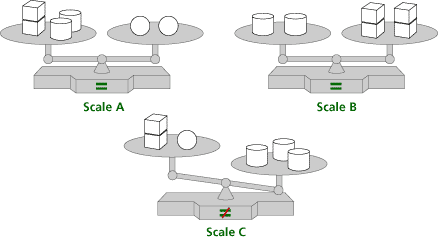a. Which block, cylinder, sphere, or cube will balance Scale C? b. List or draw the steps you followed to identify the block.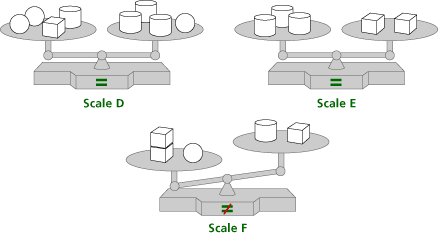c. Which block, cylinder, sphere, or cube will balance Scale F? d. List or draw the steps you followed to identify the block.

Problem D2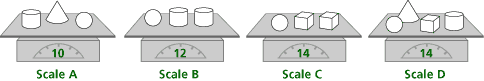a. Find the weight of each block.

cylinder = ________ pounds
sphere = ________ pounds
cube = ________ pounds

b. Write or draw the steps you followed to solve the problem.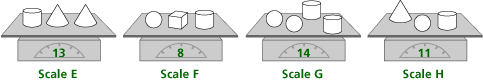c. Find the weight of each block.

cylinder = ________ pounds
sphere = ________ pounds
cube = ________ pounds

d. Write or draw the steps you followed to solve the problem.

Problem D3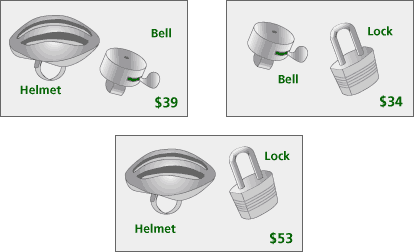These signs tell about some items for sale. Same items have same prices. Different items have different prices. How much is:

 a. A helmet? b. A bell? c. A bicycle lock? d. Explain how you figured out the prices.

Problem D4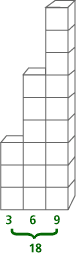This is a staircase Deion built. He used 3 blocks for the 1st stair, 6 blocks for the 2nd stair, 9 blocks for the 3rd stair, and so on, using 3 more blocks for each higher stair.

 a. Make a three-column table. In Column 1 show the Stair Number. In Column 2 show Number of Blocks used. In Column 3 show the Total Number of Blocks used to build the stairs from Stair 1 up to and including Stair 5. For example, for stairs 1-3, Deion used a total of 3 + 6 + 9, or 18, blocks. b. Let S represent the stair number, and let N represent the number of blocks. Complete this function rule to show the number of blocks needed to build Stair S. N = ? c. What is the number of blocks Deion would need to build Stair 10? d. Explain your answer to the above question.

Problem D5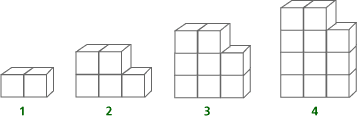This is a series of growing shapes. Imagine that the building pattern continues. How many blocks will it take to build:

 a. Shape 8? b. Shape 12? c. Tell in words how you would build Shape 5. d. Write a function rule to relate the number of blocks in a shape to the shape number. Let N represent the shape number. Let B represent the number of blocks in a shape. B = ?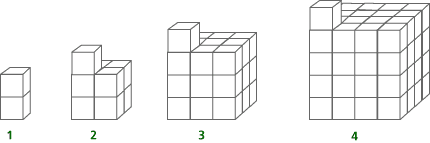This is a series of growing cubes, with one small cube on top. Imagine the building pattern continues. How many blocks will it take to build:

 e. Cube 7? f. Cube 20? g. Tell in words how you would build Cube 5. h. Write a function rule to relate the number of blocks in a cube to the cube number. Let N represent the cube number. Let B represent the number of blocks in a shape. B = ?

### Notes

Note 7

The problems in this section, while preparing students for work in more formal algebra courses, demonstrate more informal approaches to algebraic concepts, with respect to their contexts and the models they employ. Work on the problems from Groundworks,recording answers to questions (a)-(f) as you do each problem. It is important that you identify the mathematical content in each of the problems and how that content illustrates algebraic thinking.

### Solutions

Problem D1

For the first set of scales the answer is 1 cube on the left pan. For the second set of scales the answer is 1 cylinder on the right pan

a. The mathematical content is balance, the notion that the objects on the lower pan on a pan balance weigh more than the objects on the higher pan, and that one object that balances with two or more objects is the heaviest. This prepares students for understanding equality as balance and the notion that adding or removing the same items from both sides of a balance retains balance.
b. This content introduces equality as balance, setting the stage for solving equations by manipulating them while maintaining balance.
c. This content illustrates methods for solving linear equations.
d. Students approach these problems in several ways:

 • From Scale B, 2 cubes balance 1 cylinder. • Replace the 2 cylinders on Scale A with 4 cubes. • Then 2 spheres balance 6 cubes. • That means 3 cubes balance 1 sphere. • Replace the sphere on Scale C with 3 cubes. That gives 5 cubes on the left. • The 3 cylinders on the right need 6 cubes. To balance the scale, add 1 more cube.
e. To extend students’ understanding, ask questions such as the following:

 • Look at Scale A. Which block weighs the most? How do you know? • Look at Scale B. Which weighs more, a cylinder or a cube? • How many cubes balance 1 cylinder? How do you know?
f. Answers will vary. Very few textbooks contain problems of this type.

Problem D2

For the first set of scales the answers are: cylinder = 3, cube = 4,
sphere = 6, cone = 1.
For the second set of scales the answers are: cylinder = 5, cube = 1,
sphere = 2, cone = 4.

Possible responses to the questions to consider:

a. The mathematical content is the concept of variable and solving four equations in four variables.
b. This content prepares students for understanding that they can replace a variable with its value.
c. This content illustrates methods for solving systems of linear equations.
d. Students approach these problems in several ways. One way is shown for each problem.

 • For the first set of scales: Compare Scales A and D. Replace the cylinder, cone, and sphere on Scale D with 10 pounds. That means that the cube weighs 4 pounds (14 – 10). Replace the 2 cubes on Scale C with 8 pounds (2 * 4). That means the sphere weighs 6 pounds (14 – 8). Replace the sphere on Scale B with 6 pounds. Then the 2 cylinders weigh 6 pounds (12 – 6), and 1 cylinder weighs 3 pounds (6 ÷ 2). Then on Scale A, replace the cylinder and the cube with 9 pounds (6 + 3), to show the cone weighs 1 pound (10 – 9). • For the second set of scales: From Scale G, if 2 spheres and 2 cylinders weigh 14 pounds, then 1 sphere and 1 cylinder weigh 7 pounds (14 ÷ 2). Replace 1 cylinder and 1 sphere on Scale H with 7 pounds to show that 1 cone weighs or 4 pounds (11 – 7). Replace 1 cylinder and 1 sphere on Scale F with 7 pounds to show that 1 cube weighs 1 pound (8 – 7). Replace 2 cones on Scale E with 8 pounds (4 + 4) to show that 1 cylinder is 5 pounds (13 – 8). Since we know that 1 cylinder and 1 sphere weigh 7 pounds, then 1 sphere is 2 pounds (7 – 5).
e. To extend students understanding, ask them to describe other ways to solve the problems and ask questions, such as the following about the first set of scales:

 • If each cylinder on Scale B weighs 5 pounds, how much does the sphere weigh? How do you know? • If the sphere on Scale C weighs 4 pounds, how much does each cube weigh? How do you know? • Compare Scales A and D. How much does the cube weigh? How do you know?
f. Answers will vary. Very few textbooks contain problems of this type.

Problem D3

Answer: helmet = \$29, bell = \$10, lock = \$24

Possible responses to the questions to consider:

a. The mathematical content is the concept of variable and solving three equations in three variables.
b. This content prepares students for understanding that they can replace a variable with its value.
c. This content illustrates methods for solving systems of linear equations.
d. Students approach these problems in several ways. Two ways are shown.

 • Because the first two pictures each show a bell, and the 1st price is \$5 more than the 2nd, we know that the helmet costs \$5 more than the lock. In the 3rd picture, the helmet and lock together cost \$53. If the helmet was \$5 cheaper, the two would cost the same, and the combined price would be\$48 (53 – 5). Then each item would cost \$24 (48 ÷ 2). Thus, the lock costs \$24 and the helmet costs \$29 (24 + 5). Because the helmet and bell together cost \$39, the bell is \$10 (39 – 29). • Adding all the pictures together shows that 2 helmets and 2 locks and 2 bells would cost 39 + 34 + 53, or \$126. Because 2 of each item costs \$126, then 1 of each item would cost \$63 (126 ÷ 2). The 1st picture shows that the helmet and bell cost \$39, so the lock must cost \$24 (63 – 39). Similarly, the helmet costs \$29 (63 – 34), and the bell costs \$10 (63 – 53).
e. To extend students’ understanding, ask questions such as the following:

 • How are the first two pictures the same? • How are they different? • What causes the difference? • How does this difference help you solve the 3rd picture?
f. Answers will vary. Very few textbooks contain problems of this type.

Problem D4

Answers: 1, 3, 3; 2, 6, 9; 3, 9, 18; 4, 12, 30, 5, 15, 45
The rule for the number of blocks for stair N is N = 3S, so for Stair 10 it takes 3 * 10, or 30 blocks.

Possible responses to the questions to consider:

 a. The mathematical content is function. b. This content prepares students for making tables of values to represent data and for writing functions from those tables. c. This content illustrates the representation of a function as a scenario, a table of values, and an equation. d. Students approach these problems in several ways. One way is shown here. Each stair requires 3 more blocks than the prior stair. The 1st stair requires 3 blocks, the 2nd stair requires 2 * 3 blocks, the 3rd stair requires 3 * 3 blocks, so the Sth stair will require 3 * S blocks. e. To extend students’ understanding, ask them to find the rule for the total number of blocks (T) needed to build S stairs. (That rule is T = (3 / 2)S(S + 1).) f. Answers will vary. Very few textbooks contain problems of this type.

Problem D5

For the first set of shapes the rule is B = 2 + 3(N – 1) = 2N + (N – 1) = 3N – 1
For the second set of shapes the rule is B = 1 + N3

Possible responses to the questions to consider:

a. The mathematical content is inductive reasoning and writing functions.
b. This content prepares students to identify, continue, and generalize patterns.
c. This content illustrates the difference between linear and nonlinear functions.
d. Students approach these problems in several ways. One way is shown here:

 • For the first set of shapes: The 1st shape uses 2 blocks. The 2nd shape uses 2 blocks + 1 set of 3 blocks. The 3rd shape uses 2 blocks + 2 sets of 3 blocks. The Nth shape will use 2 blocks + (N – 1) sets of 3 blocks. • For the second set of shapes: The 1st shape uses a 1 * 1 * 1 cube of 1 block with 1 block on top. The 2nd shape uses a 2 * 2 * 2 cube of 8 blocks with 1 on top. The 3rd shape uses a 3 * 3 * 3 cube of 27 blocks with 1 on top. The Nth shape uses a N * N * N cube of N3 blocks with 1 on top.
e. To extend students’ understanding, ask questions such as the following:

 • How would you build the 9th or 10th or 12th shape? • How does telling how to build the shape help you write the function rule? • Describe how to build each shape in problem the first set of shapes so that you produce each of the different function rules shown in the answers.
f. Answers will vary. Very few textbooks contain problems of this type.